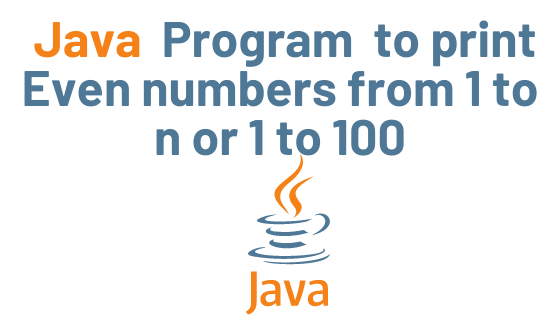## Java Program to print Even numbers with Example

In this tutorial, we will write a Java program to display even numbers from 1 to n, which means that if n is 100, this program will display the even numbers from 1 to 100.

Example: Program to display even numbers from 1 to n where n is 100

In the following example, we are displaying the even numbers from 1 to n, with the value of n set to 100, so this program will print the even numbers from 1 to 100.

An even number is one that is exactly divisible by two (never a fraction number) and yields no remainder when divided by two. This is the same logic we used to find even numbers. We are looping through 1 to n and checking each value to see if it is evenly divisible by 2; if it is, we display it.

```class JavaExample {
public static void main(String args[]) {
int n = 100;
System.out.print("Even Numbers from 1 to "+n+" are: ");
for (int i = 1; i <= n; i++) {
//if number%2 == 0 it means its an even number
if (i % 2 == 0) {
System.out.print(i + " ");
}
}
}
}```

Output:

Even Numbers from 1 to 100 are: 2 4 6 8 10 12 14 16 18 20 22 24 26 28
30 32 34 36 38 40 42 44 46 48 50 52 54 56 58 60 62 64 66 68 70 72 74 76
78 80 82 84 86 88 90 92 94 96 98 100

Java Basic Syntax | Java Tutorial

binary to decimal conversion binary to decimal conversion binary to decimal conversion binary to decimal conversion binary to decimal conversion binary to decimal conversion

ASCII is a number basedASCII is a number based ASCII is a number based ASCII is a number based ASCII is a number based ASCII is a number based

In Java, encapsulation is a mechanism for combining variables (data) and methods (code) into a single unit. It is the process of concealing information details and safeguarding the object’s data and behavior. It’s one of the four key concepts in OOP. Because the encapsulating class is simple to test, it’s ideal for unit testing.In Java, encapsulation is a mechanism for combining variables (data) and methods (code) into a single unit. It is the process of concealing information details and safeguarding the object’s data and behavior. It’s one of the four key concepts in OOP. Because the encapsulating class is simple to test, it’s ideal for unit testing.In Java, encapsulation is a mechanism for combining variables (data) and methods (code) into a single unit. It is the process of concealing information details and safeguarding the object’s data and behavior. It’s one of the four key concepts in OOP. Because the encapsulating class is simple to test, it’s ideal for unit testing.In Java, encapsulation is a mechanism for combining variables (data) and methods (code) into a single unit. It is the process of concealing information details and safeguarding the object’s data and behavior. It’s one of the four key concepts in OOP. Because the encapsulating class is simple to test, it’s ideal for unit testing.In Java, encapsulation is a mechanism for combining variables (data) and methods (code) into a single unit. It is the process of concealing information details and safeguarding the object’s data and behavior. It’s one of the four key concepts in OOP. Because the encapsulating class is simple to test, it’s ideal for unit testing.In Java, encapsulation is a mechanism for combining variables (data) and methods (code) into a single unit. It is the process of concealing information details and safeguarding the object’s data and behavior. It’s one of the four key concepts in OOP. Because the encapsulating class is simple to test, it’s ideal for unit testing.In Java, encapsulation is a mechanism for combining variables (data) and methods (code) into a single unit. It is the process of concealing information details and safeguarding the object’s data and behavior. It’s one of the four key concepts in OOP. Because the encapsulating class is simple to test, it’s ideal for unit testing.In Java, encapsulation is a mechanism for combining variables (data) and methods (code) into a single unit. It is the process of concealing information details and safeguarding the object’s data and behavior. It’s one of the four key concepts in OOP. Because the encapsulating class is simple to test, it’s ideal for unit testing.In Java, encapsulation is a mechanism for combining variables (data) and methods (code) into a single unit. It is the process of concealing information details and safeguarding the object’s data and behavior. It’s one of the four key concepts in OOP. Because the encapsulating class is simple to test, it’s ideal for unit testing. sum the elements of an array sum the elements of an array sum the elements of an array sum the elements of an array sum the elements of an array sum the elements of an array sum the elements of an array sum the elements of an array sum the elements of an array sum the elements of an array sum the elements of an array sum the elements of an array convert a char array convert a char array convert a char array vv convert a char array vconvert a char array